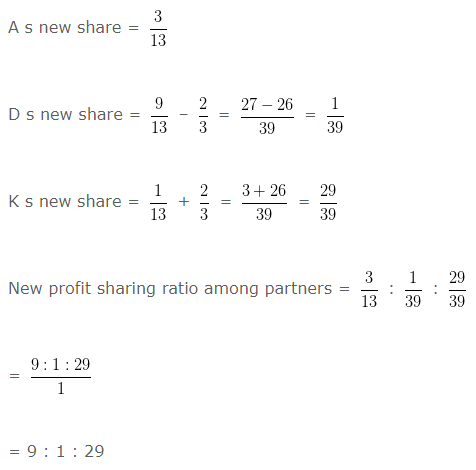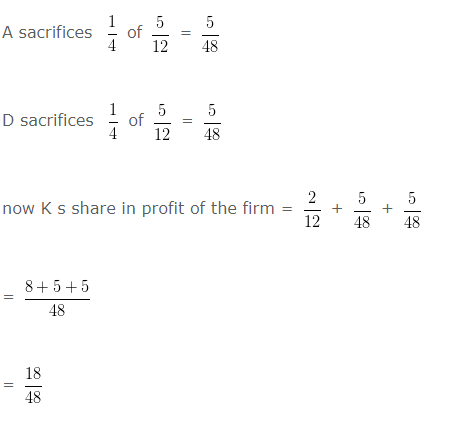# New Profit Sharing Ratio | Class 12

New profit sharing ratio is the ratio of profits amongst the partners, which arise when there is a  change in the existing profits proportion of the partners. Either there is a change in gaining ratio or change in sacrificing ratio of the partners. It is not necessary that a new profit ratio arises only in the case of admission or retirement of partner . It can also arise   in the case of change in profit sharing ratio among the partners by mutual consent or in terms of the pre-existing agreement.

The Key features of the new profit  ratio are as under : –

• It is related to all the partners including new partner.
•  New profit  ratio =  Old Ratio – Sacrificing Ratio

## New Profit sharing ratio Formulae :

• When new partner’s share is given : –  Old ratio of concerned partner × Remaining share of old partners out of total share
• When new partner acquires/takes/purchases his share from old partners: Old ratio – Sacrificing ratio of old partners
• When old partners give/surrender a part of their share in favour of new partner –  Old ratio – Surrender value of old partners

This Video computes the new profit sharing ratio amongst the partners when only profit of new partner  is given

### New Profit Sharing Ratio – Example 1

A , D and K are partners sharing losses / otherwise in the ratio of 3 , 9 and 1 respectively. K acquires 2/3 share from D . What will be the New profit ratio among partners will be:

Explanation : –### New Profit Sharing Ratio – Example 2

A , D and K are partners sharing profits and losses in the ratio of 5 : 5 : 2 respectively. A sacrifices 1/4 of his share  and D sacrifices 1/4 of his share in favour of K . What will be the sacrificing ratio between partners.

Explanation : –= 15 : 15 : 18

Sacrificing ratio = Old ratio – New ratio

A =5/12 – 15/48

= (20-15)/48

= 5/48

D = 5/12 – 15/48

= (20-15)/48

= 5/48

5 : 5

### New Profit Sharing Ratio – Example 3

F , G and H are partners sharing profits and losses in the ratio of 1 : 3 : 3 H retired from the firm and
surrendered 1/3rd of his share of prot to F and remaining in favour of G . Calculate the new profit sharing ratio of F
and G .

Explanation : –

Old ratio between F , G and H = 1 : 3 : 3

Share surrendered by H in favour of F = (3/7) x (1/3) = 3/21

Share surrendered by H in favour of G = (3/7) – (3/21)

= (9-3)/21

= 6/21

New share of F = (1/7) + (3/21)

= (3+3)/21

= 6/21

New share of G = (3/7) + (6/21)

= (9+6)/21

= 15/21

New ratio = 6/21 : 15/21

= 6 : 15

### New Profit Sharing Ratio – Example 4

G , H and I are partners sharing profit and losses in the ratio of 3 : 2 : 1. I retired from the firm and
surrendered 1/4th of his share of prot to G and remaining in favour of H . Calculate the new profit sharing ratio of G and H .

Explanation : –

Old ratio between G , H and I = 3 : 2 : 1

Share surrendered by I in favour of G = (1/6) x (1/4) = 1/24

Share surrendered by I in favour of H = (1/6) – (1/24)

= (4-1)/24

= 3/24

New share of G = (3/6) + (1/24)

= (12 + 1)/24

= 13/24

New share of H = (2/6) + (3/24)

= (8+3)/24

= 11/24

New Ratio = 13/24 : 11/24

= 13 : 11

### New Profit Sharing Ratio – Example 5

D , E and F are partners sharing profits and losses in the ratio of 1/8 : 3/8 : 4/8 F died and 1/2 of his share is taken over by D and remaining by E . Calculate the new profit sharing ratio between D and E .

Explanation : –

Old ratio between D E and F = 1 : 3 : 4

Share of F taken over by D = (4/8) x (1/2) = 4/16

Share of F taken over by E =(4/8) – (4/16)

= (8-4)/16

= 4/16

New share of D = (1/8) + (4/16)

= (2+4)/16

= 6/16

New share of E = (3/8) + (4/16)

= (6+4)/16

= 10/16

New ratio = 6/16 : 10/16

= 6 : 10

Gaining ratio = The ratio in which the continuing partners acquire the outgoing (deceased ) partner share
New share – Old share

Gaining ratio between D and E = 4/16 : 4/16

= 4:4